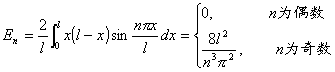### 五、二阶偏微分方程的常用解法

1.  分离变量法

它是解线性微分方程常用的一种方法，特别当区域是矩形、柱体、球体时使用更为普遍.这种方法是先求满足边界条件的特解，利用迭加原理，作这些特解的线性组合，得到定解问题的解.求特解时常归结为求某些常微分方程边值问题的特征值和特征函数.以下对不同类型方程说明分离变量法的具体解法.

[弦振动方程]

1°  两端固定的弦振动齐次方程混合问题u(x,t)=X(x)T(t)，具体解法如下：

(1)    X(x)T(t)满足的常微分方程：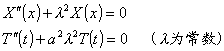(2)    用此二常微分方程的解的乘积表示弦振动方程的特解un(x,t).

解边值问题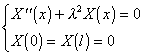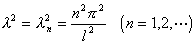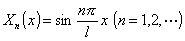λn为边值问题的特征值，Xn(x)为特征函数.λn代入T(t)的方程，得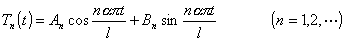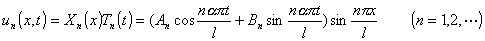(3)    un(x,t)迭加，形式上作级数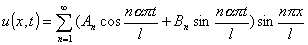(4)    利用特征函数的正交性，确定系数An,Bn.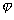(x)(x)展开成傅立叶级数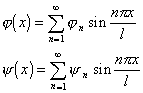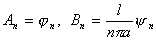(i)(x)具有一阶和二阶连续导数，三阶导数逐段连续，且(0)=(l)"(0)="(l)=0;(ii)(x)连续可微，二阶导数逐段连续，(0)=(l)=0，那末形式解右端的级数一致收敛，形式解就是混合问题的正规解.

2°  解的物理意义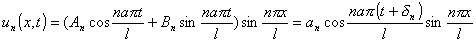3°  非齐次方程的混合问题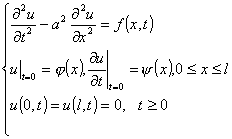u(x,t)f(x,t)展开成傅立叶级数：那末根据定解条件再利用1°(x)(x)的傅立叶展开式，有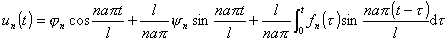(x)具有一、二阶连续导数，三阶导数逐段连续，(x)f(x,t)连续可微，二阶导数逐段连续，同时(0)=(l)="(0)="(l)=0(0)=(l)=f(0,t)=f(l,t)=0

4°  遇到非齐次边界条件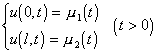[热传导方程]  热传导方程的第一边值问题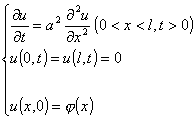u(x,t)=X(x)T(t)，得

X"(x)+2X(x)=0

T'(t)+a22T(t)=0

特征值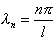，对应的特征函数为，而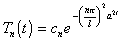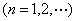作形式解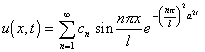(x)具有一、二阶连续导数，三阶导数逐段连续，(0)=(l)=0，则上述级数一致收敛，形式解就是正规解了.

[拉普拉斯方程]  球内定常温度分布的狄利克莱问题―拉普拉斯方程的狄利克莱问题.

选用球坐标u(r,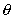,)=v(r,)().代入方程，分离变量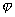`"()+k2()=0                                          (1)`
`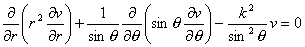(2)`

`(3)`
`(4)`

λn2=n(n+1)        (n=0,1,2,)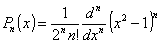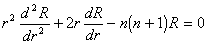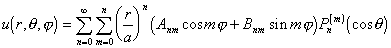如果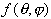二次连续可微，则表示的级数一致收敛，它就是狄利克莱问题的解.

[高阶方程]  梁的横向振动方程为

`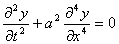（a为常数）                             (1)`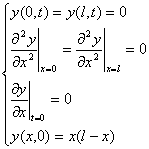y(x,t)=X(x)T(t)，那末

`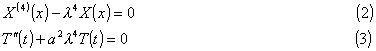`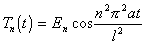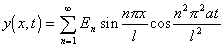y(x,0)=x(lx)